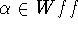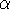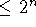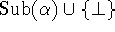Next: The Puzzle of Unfaithful Up: Kripke-type Semantics Previous: Soundness of KT5-models

## Completeness of KT5-models

As for the completeness of KT5-models, we have the following theorems.

Theorem 3. (Generalized Completeness Theorem) Any consistent set of well formed formulas is realizable.

Theorem 4. (Completeness and Decidability Theorem) For any,is a theorem of KT5 if and only ifis valid in all KT5- models whose cardinality, where n is the cardinality of the finite set.

Yasuko Kitajima
Fri Jun 20 13:39:43 PDT 1997